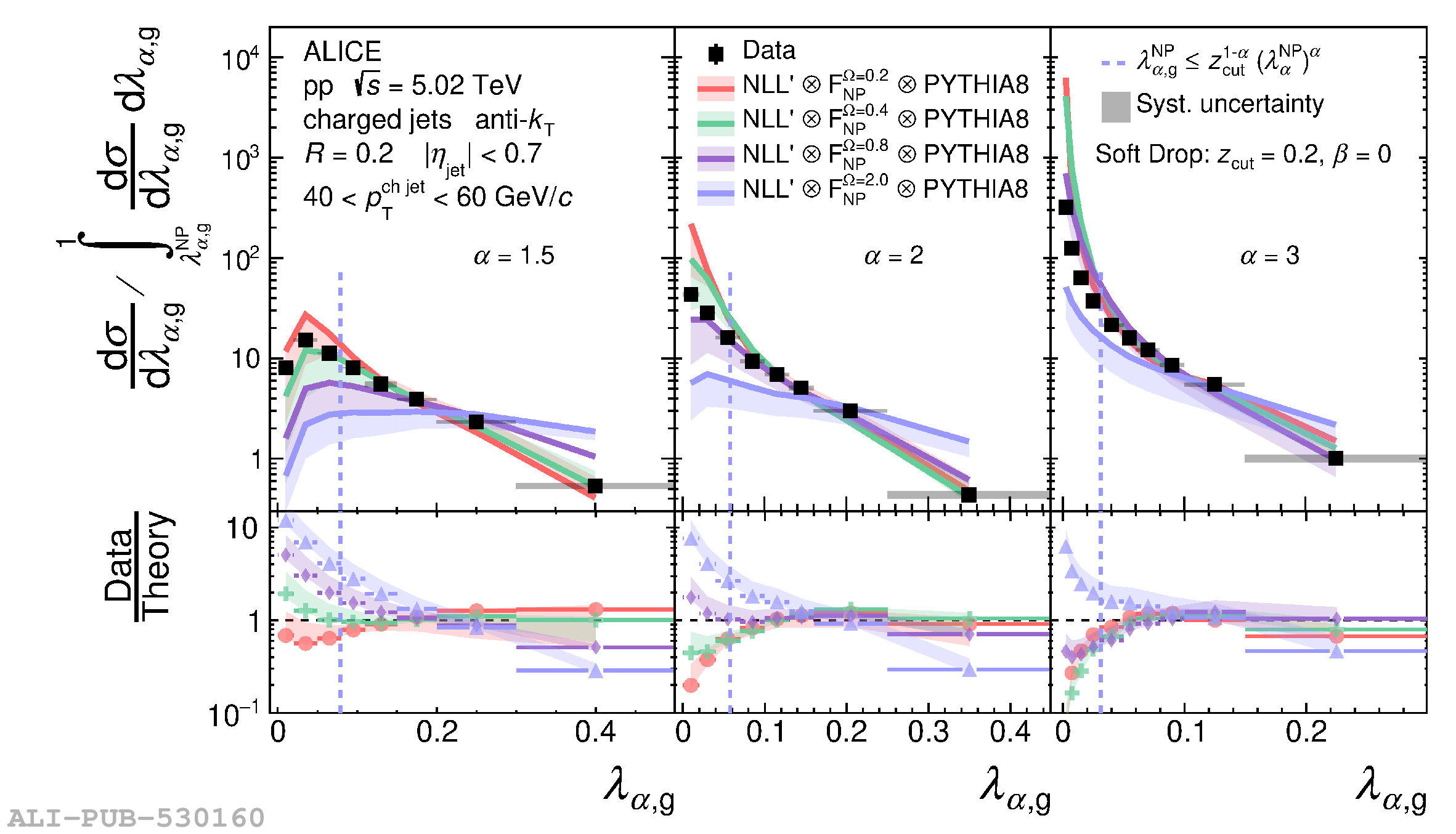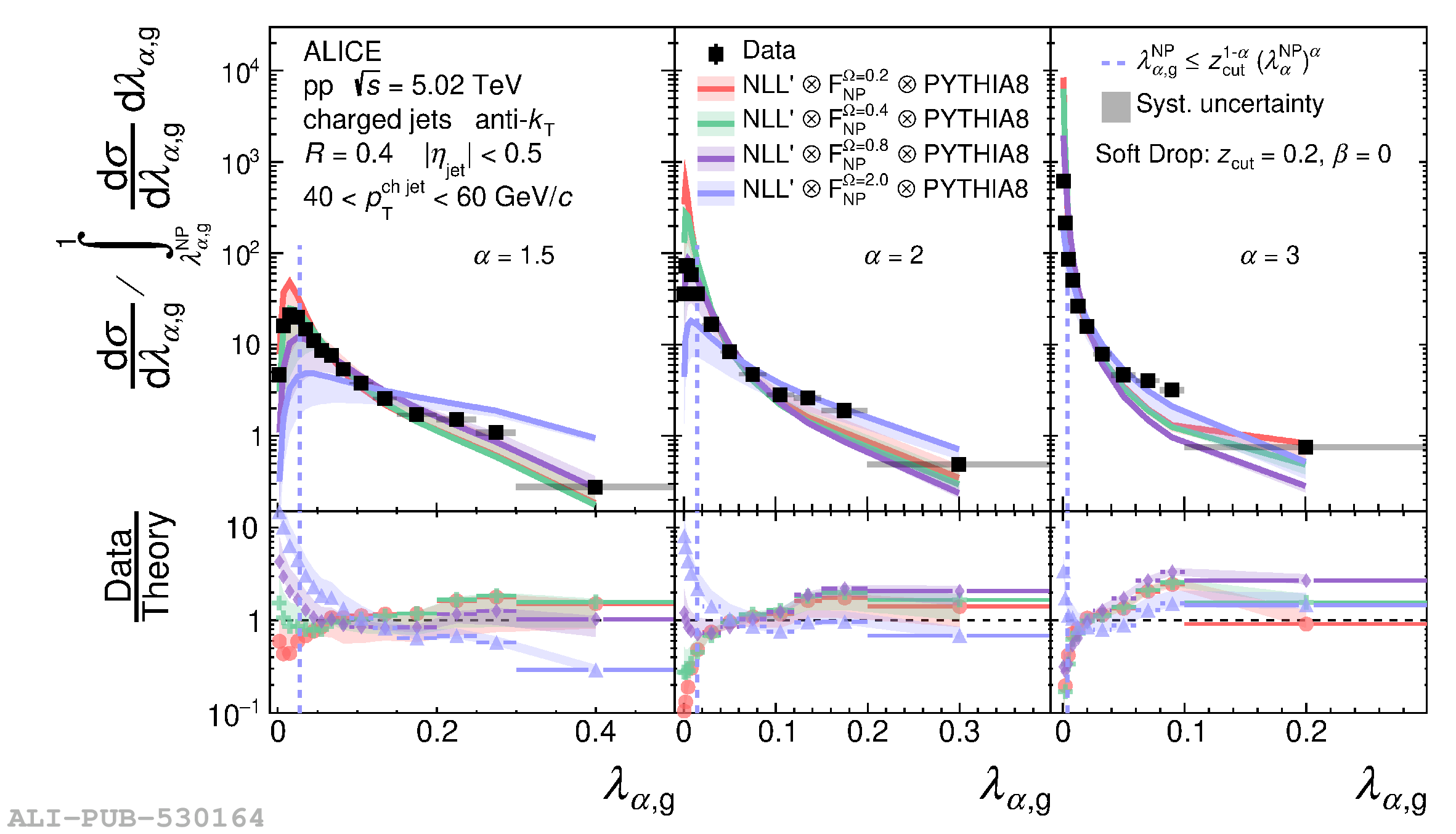# Figure A.5

 Comparison of groomed jet angularities \angsd{} in \pp{}collisions for $R=0.2$ (top) and $R=0.4$ (bottom) to analyticalNLL$^\prime$ predictions using $F(k)$ convolution in therange $40 < \pTchjet{} < 60$ \GeVc{}. The distributions arenormalized such that the integral of the perturbative region definedby $\angsd > \angsdNP{}$ (to the right of the dashed vertical line)is unity. Divided bins are placed into the left (NP) region.